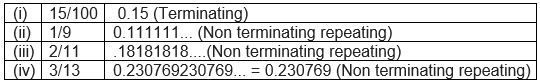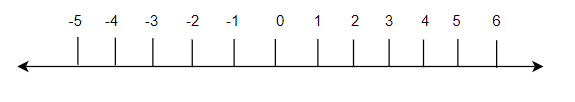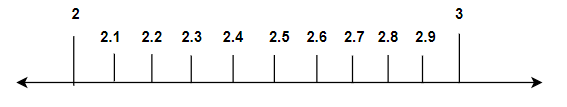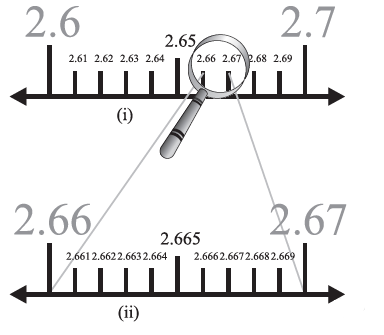# Number System Class 9 Notes

In this page we will explain the topics for the chapter 1 of Number System Class 9 Maths.We have given quality Number System Class 9 Notes along with video to explain various things so that students can benefits from it and learn maths in a fun and easy manner, Hope you like them and do not forget to like , social share and comment at the end of the page.
What is Number ( From wikipedia)

A number is a mathematical object used to count, measure, and label. The original examples are the natural numbers 1, 2, 3, and so forth. A notational symbol that represents a number is called a numeral. In addition to their use in counting and measuring, numerals are often used for labels (as with telephone numbers), for ordering (as with serial numbers), and for codes (as with ISBNs). In common usage, number may refer to a symbol, a word, or a mathematical abstraction.

In mathematics, the notion of number has been extended over the centuries to include 0, negative numbers, rational numbers such as 1/2 and -1/2, real numbers such as &radic2 , complex numbers, which extend the real numbers by including &radic(-1), and sometimes additional objects. Calculations with numbers are done with arithmetical operations, the most familiar being addition, subtraction, multiplication, division, and exponentiation. Their study or usage is called arithmetic. The same term may also refer to number theory, the study of the properties of the natural numbers.

Table of Content

## Introduction

### What is Natural Numbers?

Set of counting numbers is called the Natural Numbers
$N = {1,2,3,4,5,...}$

### What is whole number?

Set of Natural numbers plus Zero is called the Whole Numbers
$W= {0,1,2,3,4,5,....}$
Note:
So all natural Number are whole number but all whole numbers are not natural numbers

Examples:

2 is Natural Number
-2 is not a Natural number
0 is a whole number

### Integers

Lets look at what are integers
Integers is the set of all the whole number plus the negative of Natural Numbers
$Z={..,-7,-6,-5,-4,-3,-2,-1,0,1,2,3,4,5,6,..}$
Note
• So integers contains all the whole number plus negative of all the natural numbers
• the natural numbers without zero are commonly referred to as positive integers
• The negative of a positive integer is defined as a number that produces 0 when it is added to the corresponding positive integer
• natural numbers with zero are referred to as non-negative integers
• The natural numbers form a subset of the integers.

## Rational and Irrational Numbers

### Rational Number

: A number is called rational if it can be expressed in the form $\frac {p}{q}$ where p and q are integers ( q> 0).
Example : $\frac {1}{2}, \frac {4}{3},\frac {5}{7} ,1$ etc.
Important Points to Note
• every integers, natural and whole number is a rational number as they can be expressed in terms of p/q
• There are infinite rational number between two rational number
• They either have termination decimal expression or repeating non terminating decimal expression.SO if a number whose decimal expansion is terminating or non-terminating recurring then it is rational
• The sum, difference and the product of two rational numbers is always a rational number. The quotient of a division of one rational number by a non-zero rational number is a rational number. Rational numbers satisfy the closure property under addition, subtraction, multiplication and division.

Watch this tutorial for more explanation about What are rational numbers

### Irrational Number

A number is called rational if it cannot be expressed in the form p/q where p and q are integers ( q> 0).
Example : $\sqrt {3},\sqrt {2},\sqrt {5}$ etc
Important Points to Note
• Pythagoras Theorem: In a right-angled triangle, the square of the hypotenuse is equal to the sum of the squares of the other two sides. Using this theorem we can represent the irrational numbers on the number line.
• They have non terminating and non repeating decimal expression. If a number is non terminating and non repeating decimal expression,then it is irrational number
• The sum, difference, multiplication and division of irrational numbers are not always irrational. Irrational numbers do not satisfy the closure property under addition, subtraction, multiplication and division

Example
Write the following in decimal form and say what kind of decimal expansion each has:
(i) 15/100
(ii) 1/9
(iii)2/11
(iv) 3/13Example
Express the following in the form p/q where p and q are integers and q ≠ 0.
$0.\overline{7}$
$0.\overline{7}=0.7777..$ Let $x = 0.777...$
$10x = 7.777...$
$10x =7+ x$
$9x = 7$
$x = \frac {7}{9}$

## Real Numbers:

• All rational and all irrational number makes the collection of real number. It is denoted by the letter R
• We can represent real numbers on the number line. The square root of any positive real number exists and that also can be represented on number line
• The sum or difference of a rational number and an irrational number is an irrational number.
• The product or division of a rational number with an irrational number is an irrational number.
• This process of visualization of representing a decimal expansion on the number line is known as the process of successive magnification

Real numbers satisfy the commutative, associative and distributive laws. These can be stated as :
$a+b= b+a$
Commutative Law of Multiplication:
$a \times b=b \times a$
$a + (b+c)=(a+b) +c$
Associative Law of Multiplication:
$a \times (b \times c)=(a \times b) \times c$
Distributive Law:
$a \times (b + c)=(a \times b) + (a \times c)$
or
$(a + b) \times c=(a \times c) + (b \times c)$

## Laws of exponents:

A) a > 0 be a real number . m and n be integers such that m and n have no common factors other than 1, and n > 0. Then
$a^{\frac {m}{n}} =(\sqrt[n]{a})^m =\sqrt[n]{a^m}$
B) Let a > 0 be a real number and p and q be rational numbers. Then, we have
• $a^p.a^q=a^{p+q}$
• $\frac {a^p}{a^q} =a^{p-q}$
• $(a^p)^q=a^{pq}$
• $a^p.b^p=(ab)^p$

## Important Note on Irrational number expression

For positive real numbers p and q :
• $\sqrt {p} \sqrt {q}= \sqrt {pq}$
• $\sqrt {\frac {p}{q}} =\frac {\sqrt {p}}{\sqrt {q}}$
• $(\sqrt {p} + \sqrt {q})(\sqrt {p} - \sqrt {q}) =p -q$
• $(p+ \sqrt {q})(p - \sqrt {q}) = p^2 - q$
• $(\sqrt {p} + \sqrt {q})^2 = p + 2 \sqrt {pq} + q$
Note: If r is a rational number and s is an irrational number, then r+s and r-s are irrationals. Further, if r is a non-zero rational, then rs and r/s are irrationals

## What is Number Line

• A number line is a line which represent all the number. A number line is a picture of a straight line on which every point is assumed to correspond to a real number and every real number to a point
• We most shows the integers as specially-marked points evenly spaced on the line. but the line includes all real numbers, continuing forever in each direction, and also numbers not marked that are between the integers.
•• It is often used as an aid in teaching simple addition and subtraction, especially involving negative numbers.
• The number on the right side are greater than number on the left side

Each of the number explained above can be represented on the Number Line.
1. Natural Number,whole Number and integers can be easily located on the number line as we picture as per them
2. Now Real number can be either decimal expression or number explained in point 1. It is easy to located the latter one. For decimal expression, we need to use the process of successive Magnification
3. Number like $\sqrt {3}$ can be represent on number like using pythogorus theorem

## What is process of successive Magnification

Suppose we need to locate the decimal 2.665 on the Number line. Now we know for sure the number is between 2 and 3 on the number line. Now lets divide the portion between 2 and 3 into 10 equal part.Then it will represent 2.1,2.2...2.9 . Now we know that 2.66 lies between 2.6 and 2.7.Now lets divide the portion between 2.6 and 2.7 into 10 equal parts. Then these will represent 2.61,2.62,2.63,2.64,2.65,2.66...2.69. Now we know that 2.665 lies between 2.66 and 2.67 .Now lets divide the portion between 2.66 and 2.67 into 10 equal parts. Then these will represent 2.661,2.662,2.663,2.664,2.665,2.666...2.669. So we have located the desired number on the Number line. This process is called the Process of successive Magnification## True and False statement

(1) Is zero rational number? True/false
(2) Are integers rational Number ? True/false
3) Are negative number rational Numbers? True/false
4) There are infinite real numbers between 1.2 and 1.3 . True/False

### Quiz Time

Question 1 What is the value of $(\sqrt {11} - \sqrt {2} ) (\sqrt {11} + \sqrt {2})$ ?
A)$\sqrt {11} +2 \sqrt {2}$
B) 9
C) 13
D) $\sqrt {11} -2 \sqrt {2}$
Question 2 What is the value $4^{3/2}$ ?
A) 2
B) 8
C) 16
D) 1
Question 3 An irrational number is
A) Terminating decimal expression
B) Non terminating recurring decimal expression
C) Non terminating non recurring decimal expression
D) None of these
Question 4 Which is of these is false
A) The product or quotient of a non-zero rational number with an irrational number is irrational
B) Addition of two irrational number is always irrational number
C) The sum or difference of a rational number and an irrational number is irrational
D) none of these
Question 5 Who first discovered the irrational numbers?
A) Pythagorus
B) Newton
C) Archimedies
D) None of these
Question 6 Which of these is not rational number?
A) $(2+ \sqrt {2}) + (2- \sqrt {2})$
B) $\frac {11 \sqrt {5}}{2 \sqrt {5}}$
C) $\pi -2$
D) 0
Watch this Video on How to solve rational Number problem<

## Example of Simpification problems

Example -1
$(5 + \sqrt {2}) (5 - \sqrt {2})$
Solution
As
$(a + \sqrt {b}) (a - \sqrt {b})=a^2 -b$
So
$(5 + \sqrt {2}) (5 - \sqrt {2})$
$=25-2= 23$
Example -2
$( \sqrt {9} + \sqrt {2}) ( \sqrt {9} - \sqrt {2})$
Solution
As
$(\sqrt {a} + \sqrt {b}) (\sqrt {a} - \sqrt {b})=a -b$
So
$( \sqrt {9} + \sqrt {2}) ( \sqrt {9} - \sqrt {2})$
=9-2= 7

Watch this Video on How to solve Simpification problems<

## Extra Zing

Special properties around number 0 and 1

Addition Property of Zero:Adding zero to a number does not change it. For all real number $x+0=x$

Multiplication Property of Zero:Multiplying a number by zero always gives zero. For all real number $x.0=0.x=0$

Powers of Zero:The number zero, raised to any allowable power, equals zero. For n any positive number $0^n=0$ In particular, zero to the zero power ( 0n) is undefined

Zero as a numerator: Zero, divided by any nonzero number, is zero. For all real number except 0 , $\frac {0}{x}=0$ and for 0, $\frac {0}{0}$ is undefined quantity

Division by zero is not allowed: Any division problem with zero as the denominator is not defined. For example, $\frac {1}{0}.\frac {2}{0}$

Multiplication Property of One:Multiplying a number by one does not change it.For all real number $x.1=1.x=x$
Powers of One: The number one, raised to any power, equals one.For all real numbers $1^n=1$ This is true even if the n is fraction negative

Names for the number one:Any nonzero number divided by itself equals one.For all real number except 0 ,$\frac {x}{x}=1$

## Summary

Here is the Number system Class 9 Notes Summary
• Rational Numbers are those numbers which can be expressed in the p/q where p and q are integers and $q \neq 0$
• Irrational numbers are those numbers which cannot be expressed in the p/q where p and q are integers and $q \neq 0$
• Rational Numbers are terminating or non-terminating recurring and Irrational numbers are non-terminating non recurring
• Both the Rational and Irrational numbers makes the set of real numbers